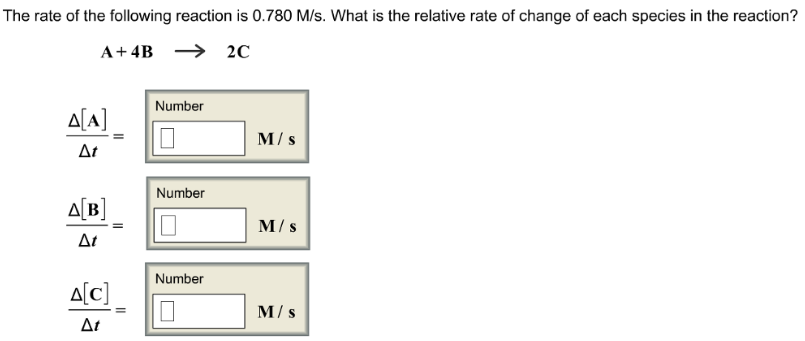# Problem: The rate of the following reaction is 0.780 M/s. What is the relative rate of change of each species in the reaction? A + 4B → 2C Δ[A]/Δt = Δ[B]/Δt = Δ[C]/Δt =

###### FREE Expert Solution
97% (89 ratings)###### Problem Details

The rate of the following reaction is 0.780 M/s. What is the relative rate of change of each species in the reaction?

A + 4B → 2C

Δ[A]/Δt =

Δ[B]/Δt =

Δ[C]/Δt =